﻿ 基于时频分析的自适应PCA辐射源调制识别
«上一篇文章快速检索 高级检索

 应用科技2018, Vol. 45Issue (5): 33-37  DOI: 10.11991/yykj.2017120130

### 引用本文GAO Jingpeng, KONG Weiyu, LIU Jiaqi, et al. Research on emitter modulation recognition of the adaptive PCA based on time-frequency analysis[J]. Applied Science and Technology, 2018, 45(5): 33-37. DOI: 10.11991/yykj.201712013.### 文章历史

1. 哈尔滨工程大学 信息与通信工程学院，黑龙江 哈尔滨 150001;
2. 试验物理与计算数学国家级重点实验室，北京 100076

Research on emitter modulation recognition of the adaptive PCA based on time-frequency analysis
GAO Jingpeng1,2, KONG Weiyu1, LIU Jiaqi2, GAO Lipeng1
1. China College of Information and Communication Engineering, Harbin Engineering University, Harbin 150001, China;
2. National Key Laboratory of Science and Technology on Test Physics & Numerical Mathematics, Beijing 100076, China
Abstract: Aiming at the problem existing in the modulation recognition such as high computational complexity and low recognition rate in the non-cooperative communication mode of a complex environment, this paper proposes an adaptive feature extraction and recognition algorithm based on time-frequency analysis. The algorithm, which combined with the second-order and fourth-order moment estimation method, uses the signal-to-noise ratio to select the principal component analysis feature adaptively, and identifies the emitter modulation method by the support vector classifier. The simulation results show that the proposed algorithm is superior to other feature extraction algorithms. When the signal-to-noise ratio is 0dB, the recognition rate is over 98%, which is about 12dB higher than that of Hu and pseudo Zernike moments. The algorithm has some advantages of a high recognition rate and a low calculated amount, having good application value in engineering application.
Keywords: emitter modulation    adaptive    principal component analysis    moment invariants    time-frequency analysis    feature extraction    support vector machines    classifier

1 系统模型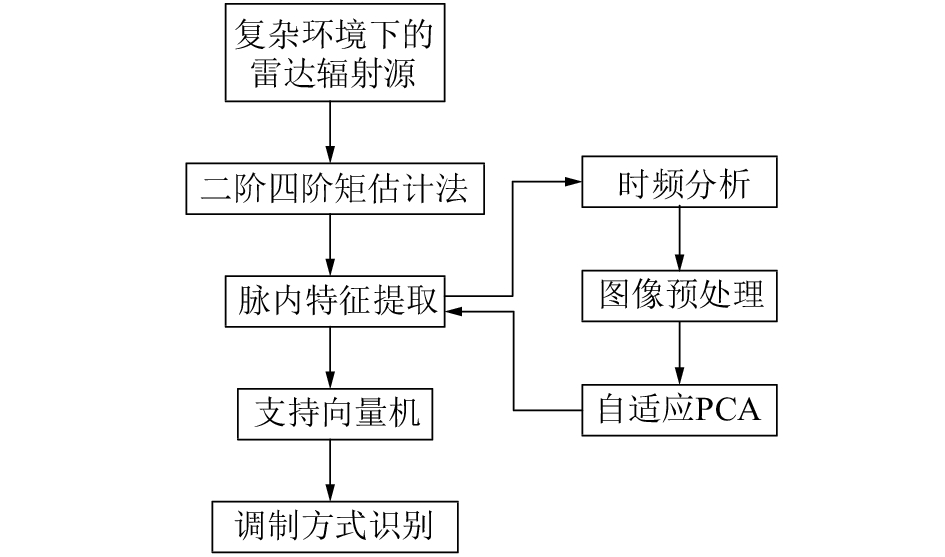Download: 图 1 系统模型

2 辐射源调制识别 2.1 时频分析

Wigner-Ville分布描述了信号的瞬时自相关函数经过傅里叶变换到时频域上的能量分布。Cohen用一个统一形式来表示一个信号z(t)的时频分布，即

 \begin{aligned}P\left( {t, \omega } \right) = & \displaystyle\frac{1}{{2\pi }}\int_{ - \infty }^\infty {\int_{ - \infty }^\infty {\int_{ - \infty }^\infty {z\left( {\mu + \frac{{\rm{ \mathsf{ \tau} }}}{2}} \right)} } } {z^*}\left( {\mu - \frac{{\rm{ \mathsf{ \tau} }}}{2}} \right) \cdot \\& \;\phi \left( {{\rm{ \mathsf{ \tau} }}, \xi } \right){{\rm{e}}^{ - {\rm{j}}\left( {t\xi + {\rm{ \mathsf{ \tau} }}f - \xi \mu } \right)}}{\rm{d}}\mu {\rm{d \mathsf{ \tau} d}}\xi =\\ & \displaystyle\frac{1}{{2\pi }}\int_{ - \infty }^\infty {\int_{ - \infty }^\infty {{\chi _z}} } \left( {{\rm{ \mathsf{ \tau} }}, \xi } \right)\phi \left( {{\rm{ \mathsf{ \tau} }}, \xi } \right){{\rm{e}}^{ - {\rm{j}}\left( {t\xi + {\rm{ \mathsf{ \tau} }}\omega } \right)}}{\rm{d \mathsf{ \tau} d}}\xi \end{aligned}

 $\begin{array}{*{20}{l}}{{W_{{\rm{CWD}}}}\left( {t,\omega } \right) = \int_{ - \infty }^\infty {{{\rm{e}}^{ - {\rm{j}}\omega {\rm{ \mathsf{ \tau} }}}}} \Biggl[\int_{ - \infty }^\infty \displaystyle{\sqrt {\frac{\sigma }{{4\pi {{\rm{ \mathsf{ \tau} }}^2}}}} z\left( {\mu + \frac{{\rm{ \mathsf{ \tau} }}}{2}} \right) \cdot } }\\{\;\;\;\;\;\;\;\;\;\;\;\;\;\;\;\;\;\;\;\;\;\;\;{z^*}\left( {\mu - \displaystyle\frac{{\rm{ \mathsf{ \tau} }}}{2}} \right){{\rm{e}}^{ - \sigma {{\left( {\mu - t} \right)}^2}/4{{\rm{ \mathsf{ \tau} }}^2}}}{\rm{d}}\mu \Biggl]d{\rm{ \mathsf{\tau} }}}\end{array}$ (1)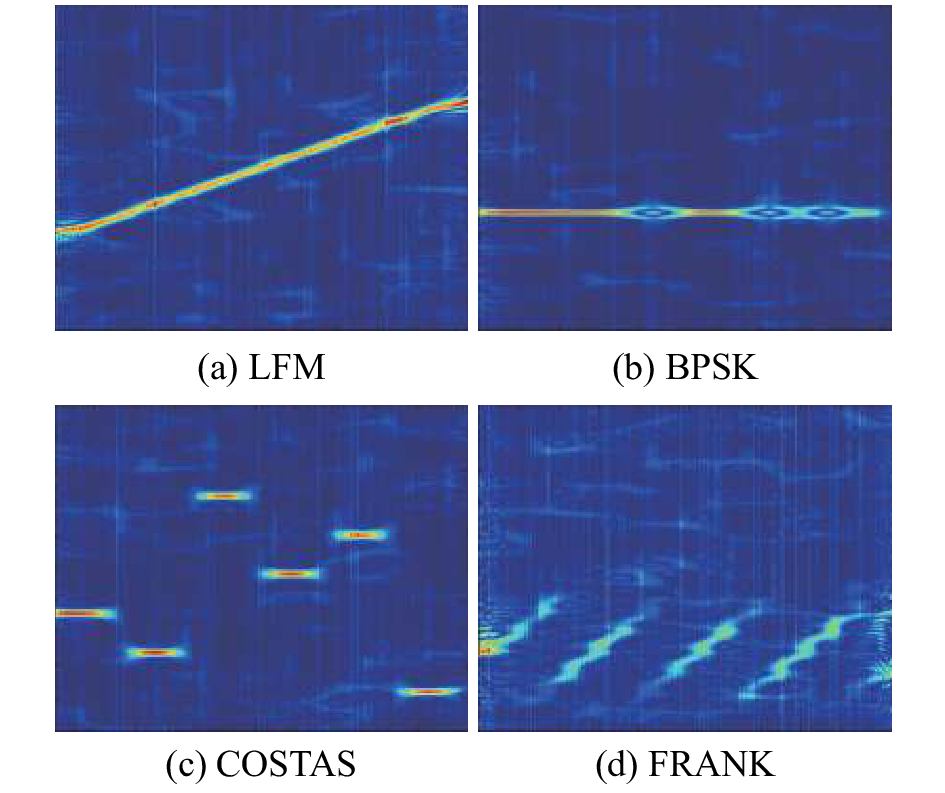Download: 图 2 4种雷达辐射源信号在信噪比为0 dB时的CWD时频图像
2.2 主成分分析算法

 ${{{ X}}_{M \times N}} = \left[ {\begin{array}{*{20}{c}}{{{{x}}_{11}}}&{{x_{12}}}& \cdots &{{x_{1N}}}\\{{x_{21}}}&{{x_{22}}}& \cdots &{{x_{2N}}}\\ \vdots & \cdots & \cdots & \vdots \\{{x_{M1}}}&{{x_{M2}}}& \cdots &{{x_{MN}}}\end{array}} \right]$ (2)

 ${{{R}}_{M \times M}} = {{U {\varLambda} }}{{{ U}}^{\rm{T}}}$ (3)

 ${{{ P}}_{M \times N}} = {{{U}}^{\rm{T}}}{{X}} = {\left[{{p_1}, {p_2}, \cdots, {p_M}} \right]^{\rm{T}}}$ (4)

2.3 二阶四阶矩估计法

x(n)二阶矩M2

 ${M_2} = {\rm E}\left[{{x_n}x_n^*} \right] = {A^2} + {\sigma ^2}$ (5)

x(n)四阶矩M4

 ${M_4} = {\rm E}\left[{{{\left( {{x_n}x_n^*} \right)}^2}} \right] = {A^4} + 2{\sigma ^4} + 4{A^2}{\sigma ^2}$ (6)

 \left\{\begin{aligned}&{{G}_{{{A}^{2}}}}=\sqrt{2M_{2}^{2}-{{M}_{4}}}\\ &{{H}_{{{\sigma }^{2}}}}={{M}_{2}}-\sqrt{2M_{2}^{2}-{{M}_{4}}}\end{aligned}\right. (7)

 ${N_{{\rm{SNR}}}} = \frac{{\sqrt {2M_2^2 - {M_4}} }}{{{M_2} - \sqrt {2M_2^2 - {M_4}} }}$ (8)

2.4 支持向量机分类器

 ${\rm{min}}\left\{ {\frac{1}{2}{w^*}w + \sum\limits_{i = 1}^n {{\xi _i}} } \right\}$

 $\begin{array}{l}L\left( {w, b, {\xi _i}, {\alpha _i}, {\lambda _i}} \right) = \displaystyle\frac{1}{2}{w^*}w + C\sum\limits_{i = 1}^n {{\xi _i}} - \sum\limits_{i = 1}^n {{\lambda _i}{\xi _i}} - \\ \;\;\;\;\;\;\;\;\;\;\;\;\;\;\;\;\;\;\;\;\;\;\;\;\displaystyle\sum\limits_{i = 1}^n {{\alpha _i}\left[{{y_i}\left( {{w_i} \cdot \varphi \left( {{x_i}} \right) + b} \right)-1 + {\xi _i}} \right]} \\ \end{array}$

 $\max \left( {a{}_i} \right) = \sum\limits_{i = 1}^n {{\alpha _i}} - \frac{1}{2}\sum\limits_{i, j = 1}^n {{\alpha _i}{\alpha _j}{y_i}{y_j}K({x_i} \cdot {x_j})}$

 $f(x) = {\rm{sgn}}\left[{\sum\limits_{i = 1}^n {{\alpha _i}{y_i}K({x_i} \cdot {x_j})} + b} \right]$

2.5 调制识别具体步骤

1）将辐射源信号代入式(5)~(8)，估计得到信号在高斯白噪声下的信噪比。

2）将辐射源信号代入式(1)，得到雷达辐射源信号的时频图像。

3）对时频图像使用灰度化、图像归一化、二值化、形态学处理等方法进行预处理，滤除冗余信息，增强有用特征。

4）利用主成分分析算法对预处理后图像提取信号特征，使用式(2)~(4)提取预处理后图像数据矩阵XM×N得到信号时频图像的骨架特征矩阵，自适应主成分分析算法依据步骤1）所得信噪比提取不同数量的主成分分量特征。

5）所有信号样本重复步骤1）~4），得到对应信噪比条件下的辐射源调制信号的样本特征数据集。

6) 将样本特征数据集的70%作为训练集，输入支持向量机，使用网格搜索法对（Cσ）2个参数寻优，以最优的（Cσ）为参数训练SVM得到分类器。

7) 将样本特征数据集的30%作为测试集，将其输入训练后的SVM分类器，进行信号调制识别。

3 仿真结果分析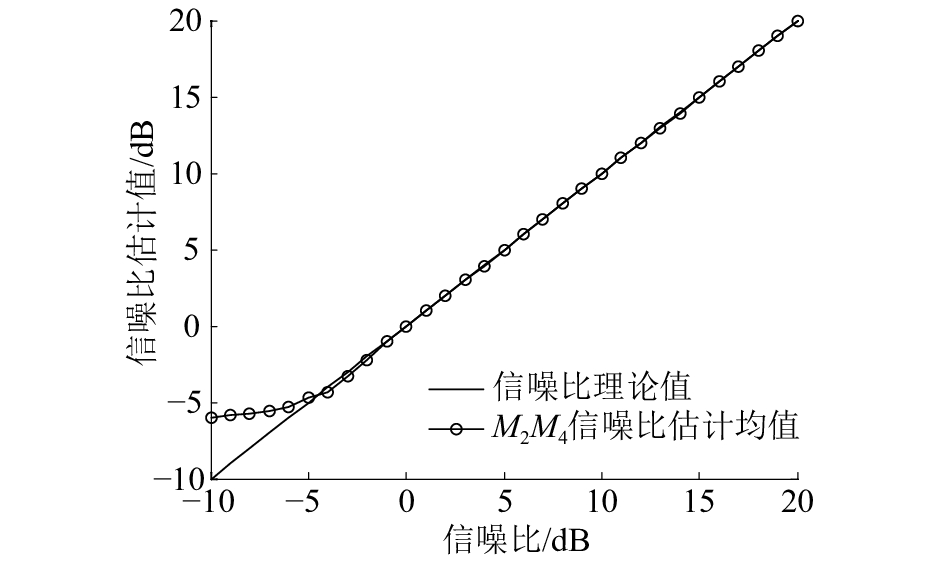Download: 图 3 二阶四阶矩估计信噪比均值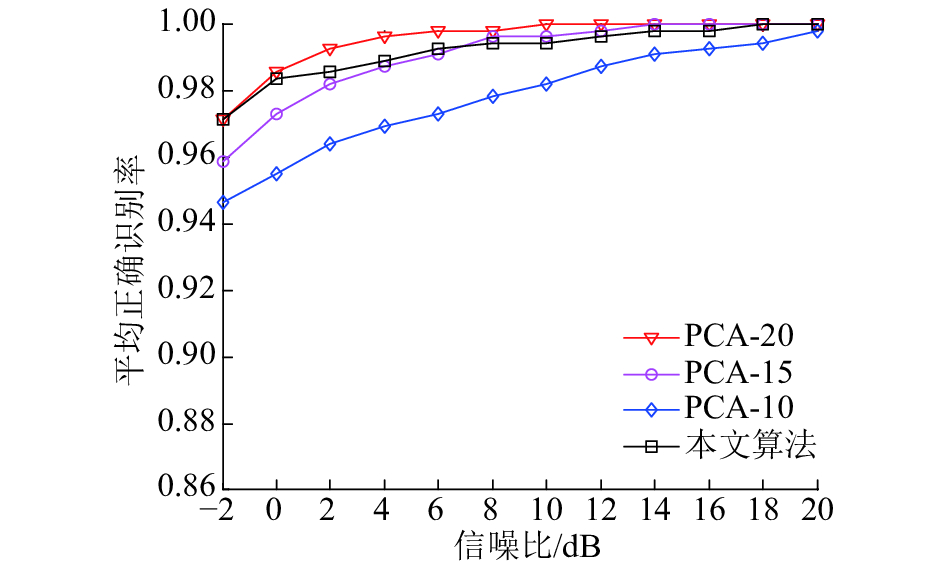Download: 图 4 自适应PCA及不同PCA特征提取识别结果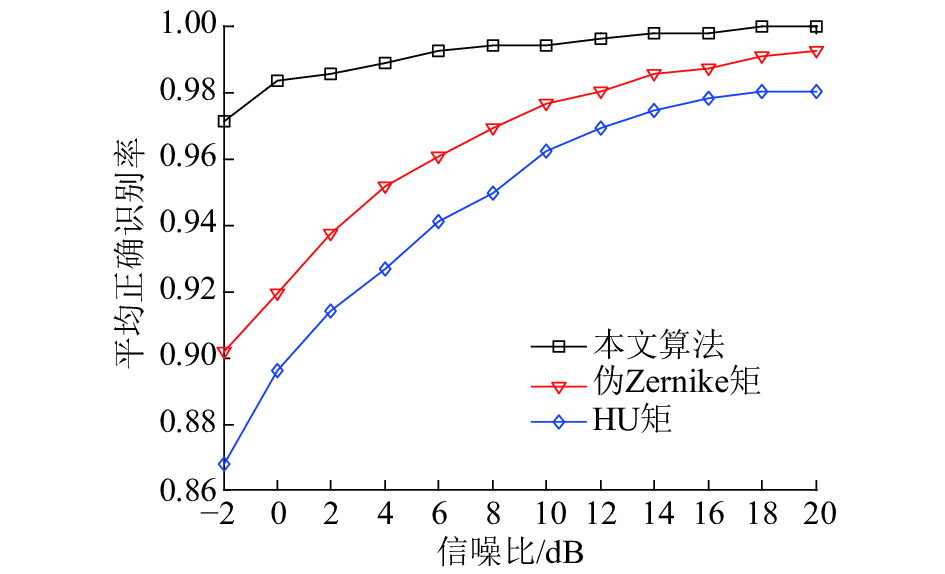Download: 图 5 自适应PCA及不变矩特征提取识别结果

4 结论

1）主成分分析算法相较于Hu矩和伪Zernike矩特征提取算法，提取雷达辐射源信号的时频图像特征能够更准确有效地应用于雷达信号调制识别。

2）将二阶四阶矩估计法与自适应PCA算法相结合，实现了在不同信噪比情况下提取不同数量的主成分分量特征。

3）自适应PCA特征提取的识别率在各个信噪比情况下都有较高的识别率，信噪比–2 dB时达到了97%以上。

4）该方法在信噪比越高时需要的主成分分量特征数越少，能够在适应不同的信噪比情况下，提升识别准确率，减少运算复杂度，具有较高的工程实践应用价值。

  ZHANG Zhenyu, HUA Zhong, LIU Yingzhe. Modulation classification in multipath fading channels using sixth-order cumulants and stacked convolutional auto-encoders[J]. IET communications, 2017, 11(6): 910-915. DOI:10.1049/iet-com.2016.0533 (0)  王静, 彭华. 多分量压缩感知信号调制参数估计算法[J]. 信息工程大学学报, 2013, 14(2): 189-195. DOI:10.3969/j.issn.1671-0673.2013.02.012 (0)  FONTES A I R, PASA L A, DE SOUSA JR V A, et al. Automatic modulation classification using information theoretic similarity measures[C]//Proceedings of 2012 IEEE Vehicular Technology Conference. Quebec City, Canada, 2012: 1-5. (0)  CAI Tian, WANG Cheng, CUI Gaofeng, et al. Constellation-wavelet transform automatic modulation identifier for M-ary QAM signals[C]//Proceedings of 2015 IEEE Annual International Symposium on Personal, Indoor, and Mobile Radio Communications. Hong Kong, China, 2015: 212-216. (0)  LI Qiang, SHEN Dong, WANG Fei. MQAM modulation recognition based on AP clustering method[C]// Proceedings of 2016 International Conference on Electronic, Information and Computer Engineering. Lanzhou, China, 2016, 44: 01002. (0)  熊坤来, 罗景青, 吴世龙. 基于时频图像和神经网络的LPI雷达信号调制识别[J]. 弹箭与制导学报, 2011, 31(5): 230-233. DOI:10.3969/j.issn.1673-9728.2011.05.066 (0)  白航, 赵拥军, 胡德秀, 等. 基于Choi-Williams时频图像特征的雷达辐射源识别[J]. 数据采集与处理, 2012, 27(4): 480-485. DOI:10.3969/j.issn.1004-9037.2012.04.014 (0)  TAVAKOLI E T, FALAHATI A. Radar Signal Recognition by CWD Picture Features[J]. International journal of communications, network and system sciences, 2012, 5(4): 238-242. DOI:10.4236/ijcns.2012.54031 (0)  LIU Yongjian, XIAO Peng, WU Hongchao, et al. LPI radar signal detection based on radial integration of Choi-Williams time-frequency image[J]. Journal of systems engineering and electronics, 2015, 26(5): 973-981. DOI:10.1109/JSEE.2015.00106 (0)  BALSUBRAMANI A, DASGUPTA S, FREUND Y. The fast convergence of incremental PCA[C]//Proceedings of the 26th International Conference on Neural Information Processing Systems. Lake Tahoe, Nevada, 2015, 2: 3174-3182. (0)  孙岩, 赵春晖, 江凌. 基于NSCT和PCA变换域的遥感图像融合算法[J]. 沈阳工业大学学报, 2011, 33(3): 308-314. (0)  于洋, 梁佳鑫, 李孟格. 标准化HU不变矩算法的车脸图像特征提取[J]. 沈阳理工大学学报, 2016, 35(6): 72-76. DOI:10.3969/j.issn.1003-1251.2016.06.014 (0)  王维平, 胡国兵. M2M4信噪比估计算法的统计性能分析[J]. 现代雷达, 2012, 34(8): 42-45. DOI:10.3969/j.issn.1004-7859.2012.08.009 (0)  魏峻. 一种有效的支持向量机参数优化算法[J]. 计算机技术与发展, 2015, 25(12): 97-100. (0)  梁礼明, 钟震, 陈召阳. 支持向量机核函数选择研究与仿真[J]. 计算机工程与科学, 2015, 37(6): 1135-1141. DOI:10.3969/j.issn.1007-130X.2015.06.015 (0)  罗小燕, 陈慧明, 卢小江, 等. 基于网格搜索与交叉验证的SVM磨机负荷预测[J]. 中国测试, 2017, 43(1): 132-135, 144. (0)